## Sunday, August 5, 2007

### Irodov Problem 1.62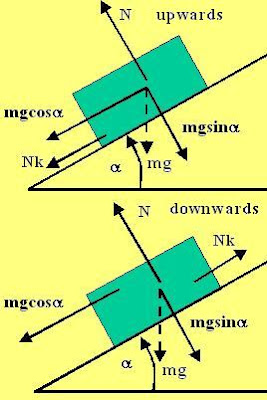First the block is pushed upwards until it stops. Suppose that it was pushed with initial velocity v and that it decelerated at a rateand that it took timeto reach the highest point before it stopped. Clearly,and thus we can calculate the distance s traveled by the block before it stops as,During the descent, say the block accelerates at a rate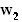and takes time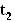to reach the base, then,Now from the problem definition we know that,. Thus, from (3)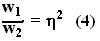Now let us determine the values of accelerations in either directions.

During Ascent:
The force diagram for the body during its ascent is depicted in the figure. We shall resolve all forces acting on the body along directions parallel and perpendicular to the inclined plane.

Along the perpendicular direction to the inclined plane there are two forces, the normal reaction from the surface N and the gravitational force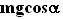. There is no acceleration in this direction and so,Along the direction parallel to the inclined plane the body experiences two forces - i) the component of force of gravity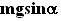and ii) the force of friction Nk opposing the upward motion and hence acting downward along the inclined plane. The body decelerates at a rate.
Thus we have,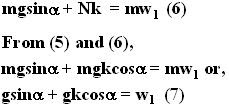During Ascent : The only different between forces acting in descent and ascent is the fact that the direction of friction force reverses since the direction of motion reverses during descent and friction force always opposes the direction of motion. Hence, during descent, the friction force acts in the upward direction along the slope. Thus,From (4), (7) and (8),#### 1 comment:

EL Fisico Juan said...

Esto es sobrenatural

Muchisimas gracias... Esto es grandioso